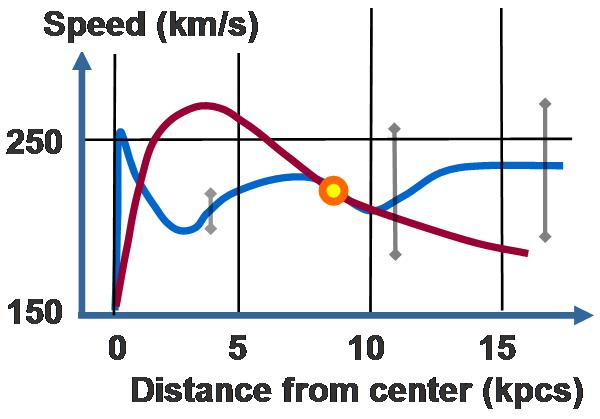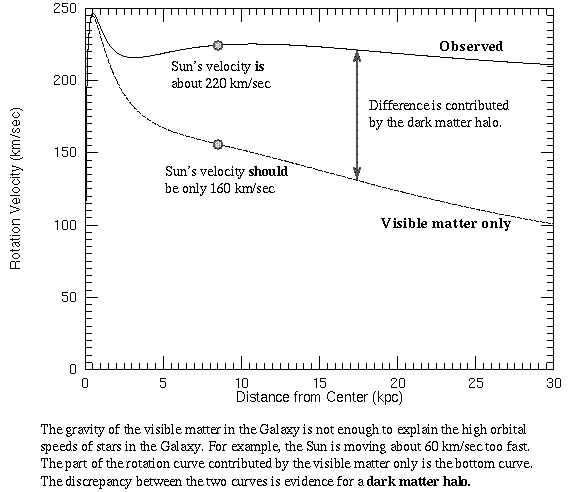# Understanding Wiki's Milky Way Galaxy rotation chart

• I
I was reading the wikipedia entry for Dark matter halo here:

https://en.wikipedia.org/wiki/Dark_matter_halo

And they have this graph for the Milky Way galaxy rotation curve:"Galaxy rotation curve for the Milky Way. Vertical axis is speed of rotation about the galactic center. Horizontal axis is distance from the galactic center. The sun is marked with a yellow ball. The observed curve of speed of rotation is blue. The predicted curve based upon stellar mass and gas in the Milky Way is red"

What I don't understand is that I found several other graphs for the Milky way rotation curve that look like this:Why in the wikipedia graph (top chart) is the predicted curve based on visible matter (red line) so different from the "visible matter only" line in the bottom graph. In the top chart the Sun sits at the intersection of the predicted (visible matter) and observed (visible + dark matter) lines.. but on the bottom graph they don't intersect at the sun. What am I missing here?

Thanks for any help with this!

Chalnoth
There's a large amount of uncertainty about precisely how much normal matter there is in the Milky Way, partially as a result of the fact that there's a lot of dust that blocks much of the light of stars further away (this is why there's a big dark band in images of the Milky Way in visible light).

So I think the best clue is the fact that in the first graph, the two points meet exactly at the location of the Sun. This likely means that they use the Sun's orbit to calculate the total mass of matter that's closer to the center of the Milky Way than we are, then assume that all of that matter is normal matter, and that it's got some particular distribution based on observations.

The graphs that show the "normal matter only" curves as much lower most likely use observational estimates of the total mass of the normal matter, rather than our orbit.

There's a large amount of uncertainty about precisely how much normal matter there is in the Milky Way, partially as a result of the fact that there's a lot of dust that blocks much of the light of stars further away (this is why there's a big dark band in images of the Milky Way in visible light).

So I think the best clue is the fact that in the first graph, the two points meet exactly at the location of the Sun. This likely means that they use the Sun's orbit to calculate the total mass of matter that's closer to the center of the Milky Way than we are, then assume that all of that matter is normal matter, and that it's got some particular distribution based on observations.

The graphs that show the "normal matter only" curves as much lower most likely use observational estimates of the total mass of the normal matter, rather than our orbit.

Yeah, that seems like a good explanation since they say it's a predicted curve based on stellar mass. It still seems odd to me that they would do this because there is such a difference in velocity in the second graph (220 km/s vs 160 km/s). You mentioned a lot of uncertainty about how much normal matter is in the Milky way. Is there enough uncertainty that some researchers still think that even without dark matter the sun would have a velocity of 220 km/s? I know that some observed galactic motion absolutely requires dark matter, but what I'm wondering is does this requirement apply at the distance of our solar system. In other words, is there any disagreement among experts that the Milky Way galaxy needs dark matter for our Sun to have its current velocity?

The second graph seems to indicate that the need for dark matter increases as the distance increases from the Galactic center.

Chalnoth